Euclidean norm

(diff) ← Older revision | Latest revision (diff) | Newer revision → (diff)

Definition

Let$R$ be a commutative unital ring. A Euclidean norm on$R$ is a function$N$ from the set of non-zero divisors of$R$ to the set of nonnegative integers, such for that for any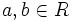$a,b \in R$ with$b$ not a zero divisor, there exist$q,r \in R$ such that: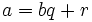$a = bq + r$

and either$r = 0$ or$N(r) < N(b)$. Such a pair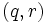$(q,r)$ is termed a quotient-remainder pair for$(a,b)$.$a$ here is the dividend and$b$ is the divisor.

For convenience, we set the norm of zero divisors as infinity.

Note that when the underlying commutative unital ring is an integral domain, zero divisor can be replaced by zero and the norm function is thus defined on all nonzero elements.

Facts

The following can be readily verified for a Euclidean norm:

• If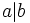$a|b$, then the norm of$b$ is at least as much as the norm of$a$.
• The units have the lowest possible Euclidean norm.

Kinds of Euclidean norms

Multiplicative Euclidean norm

A Euclidean norm is multiplicative if for any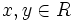$x,y \in R$,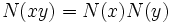$N(xy) = N(x)N(y)$.

An example of a multiplicative Euclidean norm is the usual absolute value function on the ring of integers.